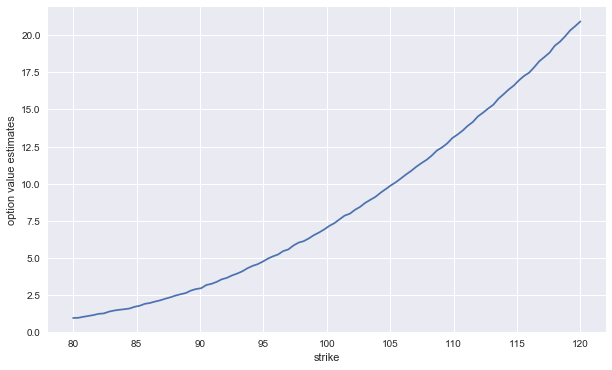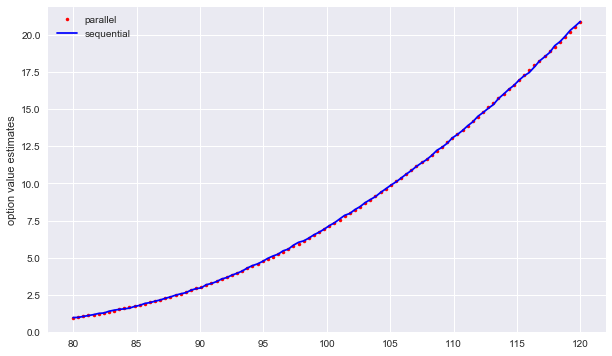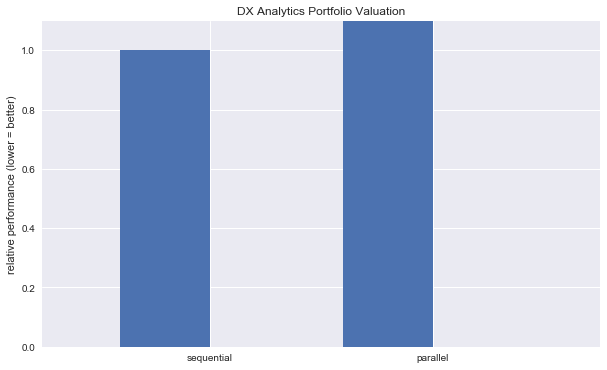# 7. Parallel Valuation of Large Portfolios¶

Derivatives (portfolio) valuation by Monte Carlo simulation is a computationally demanding task. For practical applications, when valuation speed plays an important role, parallelization of both simulation and valuation tasks might prove a useful strategy. DX Analytics has built in a basic parallelization option which allows the use of the Python mulitprocessing module. Depending on the tasks at hand this can already lead to significant speed-ups.

:

from dx import *
import time
from pylab import plt
plt.style.use('seaborn')
%matplotlib inline


## 7.1. Single Risk Factor¶

The example is based on a single risk factor, a geometric_brownian_motion object.

:

# constant short rate
r = constant_short_rate('r', 0.02)

:

# market environments
me_gbm = market_environment('gbm', dt.datetime(2015, 1, 1))

:

# geometric Brownian motion

:

# valuation environment
val_env = market_environment('val_env', dt.datetime(2015, 1, 1))

:

# add valuation environment to market environments

:

risk_factors = {'gbm' : me_gbm}


## 7.2. American Put Option¶

We also model only a single derivative instrument.

:

gbm = geometric_brownian_motion('gbm_obj', me_gbm)

:

me_put = market_environment('put', dt.datetime(2015, 1, 1))

:

am_put = valuation_mcs_american_single(
'am_put', mar_env=me_put, underlying=gbm,
payoff_func='np.maximum(strike - instrument_values, 0)')


## 7.3. Large Portfolio¶

However, the derivatives_portfolio object we compose consists of 100 derivatives positions. Each option differes with respect to the strike.

:

positions = {}
strikes = np.linspace(80, 120, 100)
for i, strike in enumerate(strikes):
positions[i] = derivatives_position(
name='am_put_pos_%s' % strike,
quantity=1,
underlyings=['gbm'],
mar_env=me_put,
otype='American single',
payoff_func='np.maximum(%5.3f - instrument_values, 0)' % strike)


### 7.3.1. Sequential Valuation¶

First, the derivatives portfolio with sequential valuation.

:

port_sequ = derivatives_portfolio(
name='portfolio',
positions=positions,
val_env=val_env,
risk_factors=risk_factors,
correlations=None,
parallel=False)  # sequential calculation


The call of the get_values method to value all instruments

:

t0 = time.time()
ress = port_sequ.get_values()
ts = time.time() - t0
print('Time in sec %.2f' % ts)

Total
pos_value    839.234
dtype: float64
Time in sec 4.09


… and the results visualized.

:

ress['strike'] = strikes
ress.set_index('strike')['value'].plot(figsize=(10, 6))
plt.ylabel('option value estimates')

:

Text(0,0.5,'option value estimates')### 7.3.2. Parallel Valuation¶

Second, the derivatives portfolio with parallel valuation.

:

port_para = derivatives_portfolio(
'portfolio',
positions,
val_env,
risk_factors,
correlations=None,
parallel=True)  # parallel valuation


The call of the get_values method for the parall valuation case.

:

t0 = time.time()
resp = port_para.get_values()
# parallel valuation with as many cores as available
tp = time.time() - t0
print('Time in sec %.2f' % tp)

Total
pos_value    840.238
dtype: float64
Time in sec 5.36


Again, the results visualized (and compared to the sequential results).

:

plt.figure(figsize=(10, 6))
plt.plot(strikes, resp['value'].values, 'r.', label='parallel')
plt.plot(strikes, ress['value'].values, 'b', label='sequential')
plt.legend(loc=0)
plt.ylabel('option value estimates')

:

Text(0,0.5,'option value estimates')### 7.3.3. Speed-up¶

The realized speed-up is of course dependend on the hardware used, and in particular the number of cores (threads) available.

:

ts / tp
# speed-up factor
# of course harware-dependent

:

0.7630898756144515

:

wi = 0.4
plt.figure(figsize=(10, 6))
plt.bar((1.5 - wi/2, 2.5 - wi/2), (ts/ts, tp/ts), width=wi)
plt.xticks((1.5, 2.5), ('sequential', 'parallel'))
plt.ylim(0, 1.1), plt.xlim(0.75, 3.25)
plt.ylabel('relative performance (lower = better)')
plt.title('DX Analytics Portfolio Valuation')

:

Text(0.5,1,'DX Analytics Portfolio Valuation')© Dr. Yves J. Hilpisch | The Python Quants GmbH